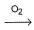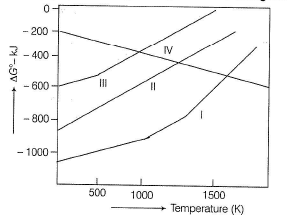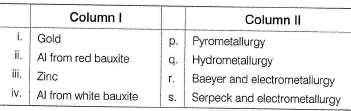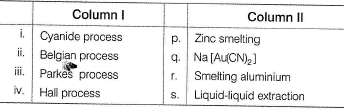Courses

# Test: Metallurgy Of Some Important Elements - II (Al, Zn, Au)

## 25 Questions MCQ Test Chemistry Class 12 | Test: Metallurgy Of Some Important Elements - II (Al, Zn, Au)

Description
This mock test of Test: Metallurgy Of Some Important Elements - II (Al, Zn, Au) for Class 12 helps you for every Class 12 entrance exam. This contains 25 Multiple Choice Questions for Class 12 Test: Metallurgy Of Some Important Elements - II (Al, Zn, Au) (mcq) to study with solutions a complete question bank. The solved questions answers in this Test: Metallurgy Of Some Important Elements - II (Al, Zn, Au) quiz give you a good mix of easy questions and tough questions. Class 12 students definitely take this Test: Metallurgy Of Some Important Elements - II (Al, Zn, Au) exercise for a better result in the exam. You can find other Test: Metallurgy Of Some Important Elements - II (Al, Zn, Au) extra questions, long questions & short questions for Class 12 on EduRev as well by searching above.
QUESTION: 1

### Only One Option Correct Type Direction (Q. Nos. 1-10) This section contains 10 multiple choice questions. Each question has four choices (a), (b), (c) and (d), out of which ONLY ONE is correct. Q.  In the electrolytic refining of zinc

Solution:

Electrodes are suspended in an electrolyte which is the solution of a soluble salt of the metal usually a double salt of the metal.

QUESTION: 2

### When compared to ΔG° for the formation of Al2O3 the ΔG° for the formation of Cr2O3 is

Solution:

ΔG° is higher for the formation of Cr2O3.

QUESTION: 3

### During the extraction of gold the following reactions take place Au + CN- + H2OX, X + Zn → Y + Au Here, X and Y respectively are

Solution:

4Au + 8KCN + O2 + 2H2O → 4K[Au(CN)2]
[4Au(CN)2]- + Zn → [Zn(CN)4]2- + Au

QUESTION: 4

Gold is extracted by hydrometallurgical process based on its property

Solution:

[Au(CN)2]- is water soluble complex.

QUESTION: 5

During Hoope’s process for electrolytic refining of Al, the middle layer is of

Solution:

In the Hoope’s process the middle layer consists of cryolite and BaF2.

QUESTION: 6

Thermite is a mixture of iron oxide and

Solution:

Thermite is a mixture of metallic oxide (Say. FeO, Cr2O3, Mn3O4) etc. and aluminium powder.

QUESTION: 7

Which is an alloy of aluminium?

Solution:

Magnalium consists of aluminium with from 5% to 30% mangesium. Duralumin is a light hard aluminium alloy containing about 4% copper and small amount of mangnesium.

QUESTION: 8

Which one of the following metals has the largest abundance in the earth’s crust?

Solution:

Aluminium is the metal which has the largest abundance in the earth's crust.

QUESTION: 9

The processes used in the refining of aluminium and zinc metals respectively are

Solution:

The processes used in the refining of aluminium and zinc metals are Hoope's process and fractional distillation.

QUESTION: 10

In sulphatizing roasting of ZnS products are

Solution:

Due to incomplete roasting ZnSO4 is formed.

QUESTION: 11

One or More than One Options Correct Type

Direction (Q. Nos. 11-15) This section contains 5 multiple choice questions. Each question has four choices (a), (b), (c) and (d), out of which ONE or MORE THAN ONE are correct.

Q.

Purification of alumina is called

Solution:

The correct answer is Option A.
The purification of alumina can be done by Baeyer's process.
In Baeyer’s process, impure bauxite is converted into pure alumina. The conversion process involves mainly three reactions:
1. Concentrated sodium hydroxide solution is heated with amphoteric bauxite at a temperature of 150 to 200°C under pressure to get sodium aluminate.
2. Sodium aluminate is then diluted with water at a temperature of 50 to 60°C. Thus, sodium aluminate gets hydrolysed to form solid aluminium hydroxide and sodium hydroxide.
3. Finally, dried aluminium hydroxide precipitate is ignited at a temperature of 1100°C to remove water molecules to get alumina.

*Multiple options can be correct
QUESTION: 12

Among the following statements the correct one is

Solution:

Argentite (silver glance). Ag2S It is an important ore of silver. Argentite are sulphide and cuprite are oxides.

*Multiple options can be correct
QUESTION: 13

The alloys having zinc metal are

Solution:

Bell metal is an alloy of copper (60% - 85%) and tin, some time including zinc or lead.
It is the type of bronze used to cost bells, German silver (Zn + Cu + Ni), Brass (Cu + Zn).

*Multiple options can be correct
QUESTION: 14

Which is a zinc compound?

Solution:

White vitriol = ZnSO4 Fool’s gold = FeS2;
Philosopher’s wool = ZnO and calomel, mercury (I) chloride

*Multiple options can be correct
QUESTION: 15

Which is not a mineral of Al?

Solution:

Spinel = MgO Al2O3, Feldspar = KAISi3O8,
Corundum Al2O3, Magnalium = Mg + Al alloy.

QUESTION: 16

Comprehension Type

Direction (Q. Nos. 16 and 17) This section contains a paragraph, describing theory, experiments, data, etc. Two questions related to the paragraph have been given. Each question has only one correct answer among the four given options (a), (b), (c) and (d).

Passage

The chief ore of zinc is ZnS.The ore is purified by floatation process and then heated in air which converts ZnS into ZnO. ZnO can be reduced with coke in Belgian process to get zinc which is purified by electrolysis.
Alternately zinc can be extracted from calamine .This after purification by levigation, subjected to calcination to get oxide. This on reduction by Belgian method followed by electrolytic refining yields pure zinc.

Q.

Zinc oxide is converted into zinc by

Solution:

Zinc oxide is converted into zinc by reduction with carbon.

QUESTION: 17

Passage

The chief ore of zinc is ZnS.The ore is purified by floatation process and then heated in air which converts ZnS into ZnO. ZnO can be reduced with coke in Belgian process to get zinc which is purified by electrolysis.
Alternately zinc can be extracted from calamine .This after purification by levigation, subjected to calcination to get oxide. This on reduction by Belgian method followed by electrolytic refining yields pure zinc.

Q.

Which of the following graph in the given figure is the Ellingham diagram of ZnO?Solution:

The third graph is the correct Ellingham diagram of Zn.

QUESTION: 18

Matching List Type

Direction (Q. Nos. 18 and 19) Choices for the correct combination of elements from Column I and Column II are given as options (a), (b), (c) and (d), out of which one is correct.

Q.

Match the Column I with Column II and mark the correct option from the codes given below.Solution:

(i) → (q), (ii) → (r), (iii) → (p), (iv) → (s)

QUESTION: 19

Match the Column I with Column II and mark the correct option from the codes given below.Solution:

(i) → (q), (ii) → (p), (iii) → (s), (iv) → (r)

*Answer can only contain numeric values
QUESTION: 20

One Integer Value Correct Type

Direction (Q, Nos. 20-24) This section contains 5 questions. When worked out will result in an integer from 0 to 9 (both inclusive).

Q.

Gibbsite formula is Al2O3 . xH2O. Here, value of x is

Solution:

Gibbsite is AI2O3.3H2O.

*Answer can only contain numeric values
QUESTION: 21

Gold is soluble in aqua-regia which is cone. HNO3 and cone. HCI in 1: x ratio. Then the value of x is

Solution:

Gold dissolves in aq ua regia i.e. HNO3 and HCI (1:3) ratio.

*Answer can only contain numeric values
QUESTION: 22

Duralumin is an important alloy of aluminium .This has 95% Al and ...... % copper.

Solution:

Duralumin is Al 95%, Cu 4%, Mg 0.5% and Mn 0.5%.

*Answer can only contain numeric values
QUESTION: 23

Al is amphoteric metal and liberate hydrogen by reacting with both with dilute acids and bases . 2 moles of Al metal when reacted with either acid or base, the number of moles of dihydrogen liberated is ......

Solution:

2 moles of Al = 6 equivalents Ai.Therefore hydrogen produced is also 6 equivalents i.e. 6g = 3 moles.

*Answer can only contain numeric values
QUESTION: 24

During the Hall-Heroult process of preparing Al metal from purified bauxite, if 3 moles of O2 is formed at anode the number of moles of Al formed at cathode is

Solution:

6O2- → 3O2 + 12e- and 4AI3+ + 12e- → 4AI.
This shows that for every 3 moles of oxygen 4 moles Al is formed.

QUESTION: 25

Statement Type

Direction (Q. No. 25) This section is based,on Statement I and Statement II. Select the correct answer from the codes given below.

Q.

Statement I : Many gem stones are impure forms of alumina (Al2O3).

Statement II : In cyanide process gold (Au) is treated with a cone, solution of sodium or potassium cyanide.

Solution:

Many gem stones are impure forms of alumina (Al2O3) for e.g. The impurity present in ruby is Cr while sapphire contains Co as the impurity. In cyanide process dilute solution (0.5%)of sodium or potassium cyanide is used.